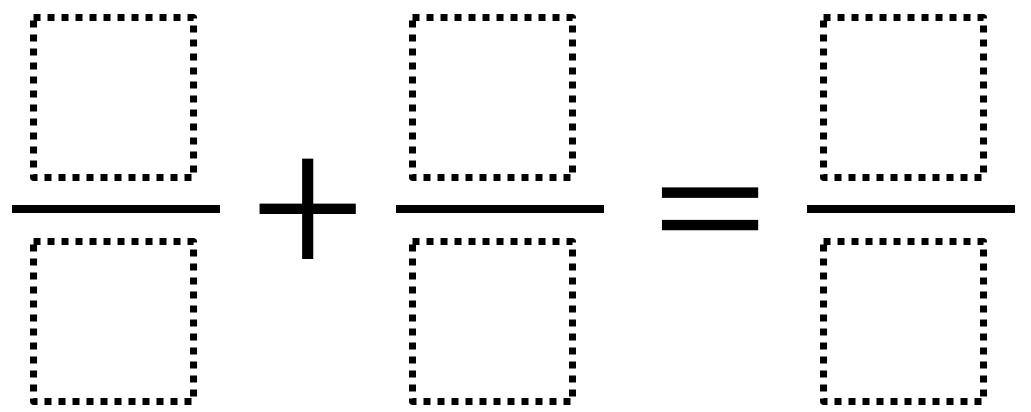Home > Grade 5 > Adding Fractions 7

# Adding Fractions 7

Directions: Use the digits 1 to 9, at most one time each, to make a true statement.### Hint

What digits would be challenging as denominators?
What digits would be challenging as numerators?

There are many answers. Here are two possibilities:
2/8 + 1/4 = 3/6
1/2 + 3/9 = 5/6

Source: Owen Kaplinsky

## Multiplying Decimals to Make a Whole Number Product

Directions: Using the digits 1 to 9, at most one time each, fill in the …

1.2.3.